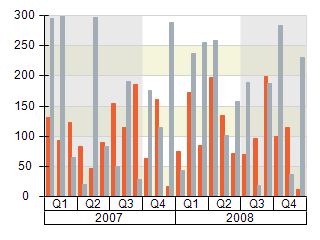﻿ Hierarchical Scale Configurator
Nevron .NET Vision Documentation
Hierarchical Scale Configurator

Hierarchical scales are useful when you have to display grouped categorical or ordinal data. Examples for such data are regional or quarterly sales report charts. In both cases you have labels that denote (or group) labels from lower levels. The following chart shows a a simple quarterly sales chart:To create a hierarchical scale you have to create an instance of the NHierarchicalScaleConfigurator class and then add some nodes to it denoting the values on the scale. The following code shows how to accomplish this:

C#
Copy Code

NChart chart = nChartControl1.Charts;

// create a hierarchical scale configuratorNHierarchicalScaleConfigurator scale = new NHierarchicalScaleConfigurator();
chart.Axis(
StandardAxis.PrimaryX).ScaleConfigurator = scale;

for (int i = 0; i < 2; i++)
{
// create a node representing the year
NHierarchicalScaleNode yearNode = new NHierarchicalScaleNode(0, (i + 2007).ToString());
yearNode.LabelStyle.TickMode =
RangeLabelTickMode.Separators;

for (int j = 0; j < 4; j++)
{
// create nodes representing the quarters within the year
NHierarchicalScaleNode quarterNode = new NHierarchicalScaleNode(3, "Q" + (j + 1).ToString());
quarterNode.LabelStyle.TickMode =
RangeLabelTickMode.Separators;
}
}

scale.CreateSeparatorForEachLevel = true;

Visual Basic
Copy Code

Dim chart As NChart = NChartControl1.Charts(0)

' create a hierarchical scale configuratorDim scale As New NHierarchicalScaleConfigurator
chart.Axis(StandardAxis.PrimaryX).ScaleConfigurator = scale

For i As Integer = 0 To 1
' create a node representing the year
Dim yearNode As New NHierarchicalScaleNode(0, (i + 2007).ToString())
yearNode.LabelStyle.TickMode = RangeLabelTickMode.Separators

For j As Integer = 0 To 3
' create nodes representing the quarters within the year
Dim quarterNode As New NHierarchicalScaleNode(3, "Q" + (j + 1).ToString())
quarterNode.LabelStyle.TickMode = RangeLabelTickMode.Separators
Next j

Next i

Scale.CreateSeparatorForEachLevel = True

Each node on the scale is represented by an instance of the NHierarchicalScaleNode class. The initializer constructor of this class accepts two arguments - the node length and text. Note that the above code sets the length of year nodes to zero. This is because it will be ignored for nodes that have children and therefore you do not have to specify it. For such nodes their length on the scale is determined by the sum of their children's length (in the above code the year node will have length of 12 as it contains four child nodes (year quarters) with length 3.

Related Examples

Windows Forms: All Examples \ Axes \ Scaling \ Hierarchical Scale

Web Forms: All Examples \ Axes \ Scaling \ Hierarchical Scale